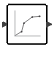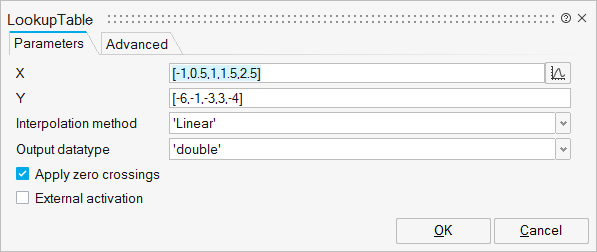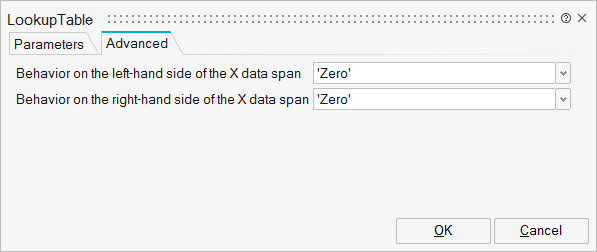# LookupTable

This block approximates a non-linear function using interpolation on data provided as a "1D-table".LookupTables

## Description

The LookupTable block approximates a non-linear function using interpolation on data provided as a "table". This is one dimensional and the table is then defined by specifying two vectors (X and Y) of size n, with x monotonic. Output of the block is the approximation of the block input using the selected interpolation method.

If Y is a matrix, then the input should be a scalar. In this case, Y and the output have the same row size.

If Y is a vector, then the input can be scalar, vector or matrix. In this case, input and the output have the same size.

## ParametersNameLabelDescriptionData TypeValid Values

xx

X

X values. Vector of doubles of size n (same size as vector Y). The X data vector must be strictly monotonically increasing. It can be either row or column provided Y is the same size.

Vector

yy

Y

Y values. Vector of doubles of size n (same size as vector X). When X is a row vector, Y can be a matrix with the same number of columns.

Matrix

Method

Interpolation method

Interpolation method, to be selected amongst: ZeroOrder(Floor), ZeroOrder(Ceil), ZeroOrder(Nearest), Linear, Not a Knot, Natural, Clamped.

String

'ZeroOrder(floor)'
'ZeroOrder(ceil)'
'ZeroOrder(nearest)'
'Linear'
'Not_a_knot'
'Natural'
'Clamped'

typ

Output datatype

Defines the datatype of the output of the block. Available datatypes are: double, int32, int16, int8, unit32, uint16, uint8.

String

'double'
'int32'
'int16'
'int8'
'uint32'
'uint16'
'uint8'
'boolean'
'inherit'

EventGen

Apply zero crossings

Enable this parameter to detect the zero crossings. Default is on.

Scalar

0
1

externalActivation

External activation

Specifies whether the block receives an external activation or inherits its activation through its regular input ports. When External Activation is selected, an additional activation port is added to the block. By default, external activation is not selected.

Number

0
1NameLabelDescriptionData TypeValid Values

Leftside

Behavior on the left-hand side of the X data span

Action that should be taken on the left-hand side of the X data.

String

'Zero'
'Hold'
'Extrapolation'
'Repeat'

Rightside

Behavior on the right-hand side of the X data span

Action that should be taken on the right-hand side of the X data.

String

'Zero'
'Hold'
'Extrapolation'
'Repeat'

## Ports

NameTypeDescriptionIO TypeNumber

Port 1

explicit

output

1

Port 2

explicit

input

1

Port 3

activation

input

externalActivation

NameValueDescription

always active

no

direct-feedthrough

yes

zero-crossing

no

mode

no

continuous-time state

no

discrete-time state

no